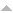# Publications

Found 248 results
Author Title [ Type] Year
Filters: First Letter Of Last Name is Y  [Clear All Filters]
Articles in international peer reviewed journals
On Abel type integral equations of first kind. J. Fract. Calc.. 1994;5:59-68.Edit
About a new class of integral transforms in Hilbert space. Math. Balkanica (N.S.). 1995;9:179-191.
Algebraic quotient modules and subgroup depth. Abh. Math. Semin. Univ. Hambg.. 2014;84:267-283.Edit
An analog of Morgan's theorem for the Kontorovich-Lebedev transform. Adv. Pure Appl. Math.. 2010;1:159-162.Edit
Analog of the Hausdorff-Young theorem for the Lebedev integral. Integral Transforms Spec. Funct.. 2005;16:597-607.
Another proof of Spira's inequality and its application to the Riemann hypothesis. Journal of Mathematical Inequalities. 2013;7(2):167-174.Edit
Another proof of Spira's inequality and its application to the Riemann hypothesis. J. Math. Inequal.. 2013;7:167-174.Edit
Applications of \$N\$-fractional calculus to infinite sums. J. Fract. Calc.. 1995;8:37-42.Edit
Asymptotic and summation formulas related to the Lebedev integrals. Integral Transforms Spec. Funct.. 2008;19:293-304.
Beurling's theorems and inversion formulas for certain index transforms. Opuscula Math.. 2009;29:93-110.
Boundedness and inversion properties of certain convolution transforms. J. Korean Math. Soc.. 2003;40:999-1014.
Central factorials under the Kontorovich-Lebedev transform of polynomials. Integral Transforms Spec. Funct.. 2013;24:217-238.
Certain identities, connection and explicit formulas for the Bernoulli and Euler numbers and the Riemann zeta-values. Analysis (Berlin). 2015;35:59-71.
Certain isometries related to the bilateral Laplace transform. Math. Model. Anal.. 2006;11:331-346.
The Cherry transform and its relationship with a singular Sturm-Liouville problem. Q. J. Math.. 2000;51:371-383.Edit
A class of index integral transforms. Rev. Técn. Fac. Ingr. Univ. Zulia. 1987;10:105-118.Edit
A class of index transforms generated by the Mellin and Laplace operators. J. Math. Anal. Appl.. 2013;403:333-343.
A class of index transforms with general kernels. Math. Nachr.. 1999;200:165-182.Edit
A class of index transforms with Whittaker's function as the kernel. Quart. J. Math. Oxford Ser. (2). 1998;49:375-394.Edit
On a class of integral convolutions. Vests\=ı Akad. Navuk Belarus\=ı Ser. F\=ız. Mat. Navuk. 1992:27-33, 124.
A class of integral equations and index transformations related to the modified and incomplete Bessel functions. J. Integral Equations Appl.. 2010;22:141-164.
On the class of Lebedev-Skalskaya type index transforms. Fukuoka Univ. Sci. Rep.. 1994;24:67-81.Edit
A class of polynomials and discrete transformations associated with the Kontorovich-Lebedev operators. Integral Transforms Spec. Funct.. 2009;20:551-567.
Closed-form evaluation of two-dimensional static lattice sums. Proc. R. Soc. A. 2016;472: 20160510.Edit
Composition theorems of Plancherel type for index transformations. Dokl. Akad. Nauk Belarusi. 1994;38:29-32, 122 (1995).

Error | CMUP

# Error

The website encountered an unexpected error. Please try again later.